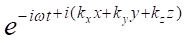top of page

# Surface plasmon polaritons

Updated: Jul 4

Plasmons: Collective oscillations of the “free electron gas density” often at optical frequencies. The coupling of light to the metal nano particles produces resonances under specific conditions. The resonant interaction between them will localize the electromagnetic field near metal surface and drastically enhances the optical scattering phenomenon. The unique properties of the interface waves result from the frequency-dependent dispersion characteristics of metallic and dielectric materials. Thus, a dielectric–metallic interface can support surface plasmon polaritons (SPP). The momentum of a surface plasmon mode is greater than the momentum of propagation light modes of same frequency. There needs to be an Ex component of the field for a surface charge to exist. Hence SPs yield TM polarized fields. The EM fields of SPs are highly confined to the metal/dielectric interface. They evanescently decrease in strength away from the interface. The tangential and normal components of the SPP wave vector have imaginary parts even in a lossless system. Consequently, the amplitude of such an SPP should decrease in the direction of propagation and increase away from the interface.

Applications of SPP

Able to concentrate energy in wavelength volume

Strong dispersive properties mechanism for manipulating waves

Able to guide waves along the surface of a metal.

Dispersion of Surface plasmon polaritons

Surface plasmon polaritons (SPPs) are quasiparticles that are formed when light couples with electrons at the interface between a metal and a dielectric material. The dispersion of SPPs refers to the relationship between the frequency and wavevector of the SPPs, and it determines the behavior of the SPPs as they propagate along the interface.

In general, the dispersion of SPPs is described by a dispersion relation, which is a mathematical equation that describes the relationship between the frequency, wavevector, and other material parameters of the system. The dispersion of SPPs is influenced by several factors, including the metal used, the dielectric material, and the thickness of the metal film.

For instance, the dispersion of SPPs can be either positive or negative, depending on the combination of metal and dielectric material used. Positive dispersion means that the frequency of the SPPs increases with increasing wavevector, while negative dispersion means that the frequency decreases with increasing wavevector.

The dispersion of SPPs is important for several applications, such as surface-enhanced spectroscopies, photonics devices, and subwavelength imaging. The understanding of the dispersion of SPPs is crucial for the design and optimization of these applications.

Derivation of the dispersion equation

This relation is the basis for understanding of coupling of light to propagating surface plasmons, by using special approaches to match the wavevector. Surface plasmon polariton is a surface wave propagating along the metal dielectric interface or it is a coupled state between a photon and plasmon.Incident Wave: E and H depends on Z-variable and time in the form of plane waveSurface wave : EM wave energy concentrated at the interface (z direction)and decay away from the interface, Surface ,are independent on Y-Amplitudes of Electric and magnetic fields along the x direction

The energy of polaritons is contributed by two parts. One part is pure EM energy accumulated in EM field and second part is energy of solid state.

Light couples with electron oscillations (Plasma oscillations in metal)

Therefore,

EM energy +kinetic energy of free electronsEnergy of resulting wave (SPP)

A plane wave has translational symmetry along z and Y directions

Without loss of generality we can assume that E and H are independent on Y. We need to find distribution of E an M Field.

I.e. Dependence of frequency of surface plasmon polariton on propagation constant kzUsing boundary conditions

We need to find dispersion equation of surface EM wave using from the fundamental Maxwell’s equations Maxwell’s equationEquivalent to 6 scalar equations

A t the interface six components of electric and magnetic field can be divided into two setsTM polariton i.e. connection between Hy, Ex, Ez component of EM fieldTE polarization, TE polarization- Ey, Hx & HzIn TM polarization only we can get non-trivial solution

Dispersion EquationHelmholtz equation for Hy component of magnetic field

Ex and Ez in terms of magnetic fieldSolution for Helmholtz equation in upper and lower medium

Upper mediumLower mediumAfter applying boundary conditions x=0,

Continuity of tangential component of magnetic field

H1y (0) =H2y (0)Continuity of tangential component of electric field

Substitute x=0 in the above equationsE1z (0) =E2z (0)k1, k2 inverse penetration depths in medium1 and medium 2 respectively.

Substitute k1 & k2, k1= k2 =positiveDispersion equation of SPPThis expression relates the wavenumber of the SPP along the propagation direction with the wavenumber of electromagnetic waves in vacuum k0. The unique properties of the interface waves result from the frequency-dependent dispersion characteristics of metallic and dielectric materials.